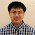## A multimeter is a tool that uses to measures electrical characteristic such as voltage, ampere, resistance. Using a multimeter and basic knowledge of ohm's law can help electronics projects much easier.

There is two type of multimeter one is a digital multimeter and another is an analog multimeter. The advantage of digital multimeter is easy to read value, lower power consumption, and higher price model can measure diode, capacitance, frequency, duty cycle, inductance, the temperature you can call it all in one multimeter instead of a multimeter.

#### How does a multimeter work?

Basic functions of multimeter are measure voltage, ampere, and resistance.
A multimeter changes electrical characteristic values to numbers for digital multimeter or meter scale for an analog multimeter.

### What type of multimeter you need:

• - If your project involves high dc current you must use high DC current type multimeter, the high current multimeter probe usually bigger and have separate high DC/AC current measure socket
• - If your project involves high AC current you must use clamp meter or clamp multimeter, clamp meter can handle up to 400 Ampere AC current without problem
• - Some multimeter model has temperature measurement function the function is good for  project that involves with temperature monitor/control
• - If you want to measure very high current and high voltage you may need specific types such as a current meter or high voltage meter.

### Symbols on a multimeter

V  = Voltage
A  = Ampere
~   = AC (Alternate current)
⎓  = DC (Direct current)
Ω  = Ohms (Resistance)
-||- = Capacitor

Diode

Before you start using a multimeter to measure voltage, make sure you select voltage range to maximum (750 V for normal multimeter) and adjust to a lower range when you need, if you have auto range digital multimeter you can forget about it.

See picture below to see manual range or auto range multimeter, a manual range multimeter has different range step but an auto range multimeter has only Voltage sign only.

### How to use a multimeter to measure DC voltage

• - rotate selector to V⎓ because battery power supply is a DC type current
• - connect a multimeter com probe (black color) to a power supply ground
• - connect a multimeter red probe to load V+
The picture below is a led current limiter using a resistor. You can see 2.708 volts between load(Led) and ground that mean voltage across the load(Led) just half of dc power supply and voltage across the resistor(use for limit current to Led) around 5.0 - 2.7 =
2.3 volts, 5 volts is the dc power supply voltage (4 ni-MH rechargeable battery 1.25 each cell)

You can find current across the resistor around V/R=I >>> 2.3(V)/1000(resistor value) = 0.0023 Ampere(I)
note:1000 mAh = 1 Ampere 2.3 mAh = 0.0023 Ampere. The circuit loss power to resistor around
P = IV 0.0023x 2.3 = 0.00529 Watt or 5.29 mW while the load(Led) consume 6.22 mW that mean the circuit efficiency very low compare to over 90% of dc to dc converter to limit voltage and current to Led.

### How to using multimeter measure Ampere

Using multimeter to measure current to load instructions
• - Change selector to mA⎓ or A⎓ if you need to measure high current load (high current version multimeter can measure up to 20 Ampere DC current or more)
• - connect Com probe(black color) to a power supply ground
• - connect red probe to load ground
• - connect load V+ to a power supply (or current limit resistor)
• Using the current value x voltage across the load to measure power consumption (watt)
• - 1000 mW = 1 watt
• - 1000 watt = 1 kilo-watt
• - Current must not exceed maximum value the multimeter can withstand or internal replaceable fuse blow out, you need to change new one with same type, voltage rate, amp rate
See the picture below for better understand

### Using multimeter to measure resistive value

• - Change selector to Ω
• - connect com probe to resistor's leg
• - connect red probe to another leg of the resistor
• - check carefully that no voltage across a resistor if you measure it in a circuit board
• the resistor use in the picture is 1 kilo-ohms value
See below picture for better understand

### Multimeter maintenance guide

• - Change batteries when low batt sign shows, the multimeter may not functions well if you don't change new batteries. Commercial multimeter use 2xAA or 9 V batteries as standard
• - Remove batteries from a multimeter battery case if you do not use it for a long time
• - check probe wire to find damage regularly and repair or change broken wire if you are dealing with high current and voltage electrical machine measurement
• - keep the multimeter out of reach of children
• - keep the multimeter in a dry and low humidity area
Enjoy hobby world for easyandworkproject.xyz

1.This comment has been removed by a blog administrator.